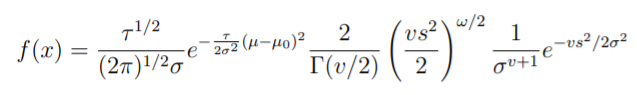# Gamma-Normal Distribution

Share on

The gamma-normal distribution (also called the Gaussian normal distribution, GN distribution or normal-gamma distribution) is a bivariate compound probability distribution for the sum of a gamma random variable and a normal/Gaussian random variable. It is the conjugate prior (from Bayesian statistics) of a normal distribution with unknown mean and precision . “Conjugate” here means that the surface is a gamma-normal both before and after historical results have been taken into account. As you collect more sample data, the parameters change and therefore the shape of the surface changes as well.

The distribution has four parameters: mu (μ), kappa (κ, alpha α, and beta β. The distribution can describe a multitude of surface shapes, depending on these four values (a.k.a. parameters).

If random variables X, Y, have a normal-gamma distribution, then the marginal distribution of Y is a gamma distribution with parameters α, β. The conditional distribution of X, given Y, is a normal distribution with expected value μ and logged standard deviation κ -log(Y)/2.

## Probability Density Function for the Gamma-Normal Distribution

If X ~ N(μ, σ2) and σ ~ inverse gamma (α, β), then the PDF of the random variable X is given by :For -∞ x ∞.

## References

 Bernardo, J.M.; Smith, A.F.M. (1993) Bayesian Theory, Wiley. ISBN 0-471-49464-X
 Theorem. Retrieved December 7, 2021 from: http://www.math.wm.edu/~leemis/chart/UDR/PDFs/NormalGammanormal.pdf

CITE THIS AS:
Stephanie Glen. "Gamma-Normal Distribution" From StatisticsHowTo.com: Elementary Statistics for the rest of us! https://www.statisticshowto.com/gamma-normal-distribution/
---------------------------------------------------------------------------Need help with a homework or test question? With Chegg Study, you can get step-by-step solutions to your questions from an expert in the field. Your first 30 minutes with a Chegg tutor is free!